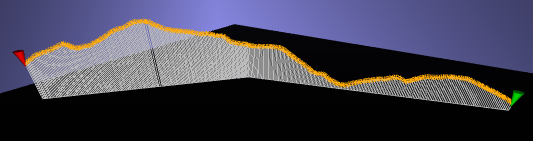# Drape lines on a DEM with PostGIS

This article gives a few SQL commands to drape 2D geometries on a DEM (Digital Elevation Model), in order to obtain 3D geometries. We use PostGIS 2, and its rasters support especially.

Assuming you have a DEM compatible with GDAL, you can easily load the raster into the database using these commands.

Reprojects to specified SRID, crops to specified extent, and writes output in a file:

```gdalwarp -t_srs EPSG:32632 -te 289942 4845809 400671 4947295 dem_file.geotif output.bin
```

Tiles into 100 pixels squares and converts to SQL:

```raster2pgsql -c -C -I -M -t 100x100 output.bin mnt > output.sql
```

```psql -d yourdb < output.sql
```## Drape geometries

There are at least 3 strategies to drape your geometries.

### With geometry resolution

We obtain one elevation value per point on your line.

Pros: You keep your original geometry resolution (number of points)

Cons: You potentially loose a lot of 3D information (think of "hops")``` WITH line AS
-- From an arbitrary line
(SELECT 'SRID=32632;LINESTRING (348595 4889225,352577 4887465,354784 4883841)'::geometry AS geom),
points2d AS
-- Extract its points
(SELECT (ST_DumpPoints(geom)).geom AS geom FROM line),
cells AS
-- Get DEM elevation for each
(SELECT p.geom AS geom, ST_Value(mnt.rast, 1, p.geom) AS val
FROM mnt, points2d p
WHERE ST_Intersects(mnt.rast, p.geom)),
-- Instantiate 3D points
points3d AS
(SELECT ST_SetSRID(ST_MakePoint(ST_X(geom), ST_Y(geom), val), 32632) AS geom FROM cells)
-- Build 3D line from 3D points
SELECT ST_MakeLine(geom) FROM points3d;
```

Note by Daniel Gerber: if the line goes outside your DEM, use a left join (FROM points2d LEFT OUTER JOIN elevation ON ST_Intersects(...)) and set default value to 0.0 with coalesce(ST_Value(..), 0.0).

### With DEM resolution

We obtain one elevation value per cell of your raster.

Cons: You may increase tremendously the resolution of geometries``` WITH line AS
-- From an arbitrary line
(SELECT 'SRID=32632;LINESTRING (348595 4889225,352577 4887465,354784 4883841)'::geometry AS geom),
cells AS
-- Get DEM elevation for each intersected cell
(SELECT ST_Centroid((ST_Intersection(mnt.rast, line.geom)).geom) AS geom,
(ST_Intersection(mnt.rast, line.geom)).val AS val
FROM mnt, line
WHERE ST_Intersects(mnt.rast, line.geom)),
-- Instantiate 3D points, ordered on line
points3d AS
(SELECT ST_SetSRID(ST_MakePoint(ST_X(cells.geom), ST_Y(cells.geom), val), 32632) AS geom
FROM cells, line
ORDER BY ST_Distance(ST_StartPoint(line.geom), cells.geom))
-- Build 3D line from 3D points
SELECT ST_MakeLine(geom) FROM points3d;
```

### Sampling

We obtain one elevation value per step of X units (meters).

Pros: You control the resulting resolution

Cons: Sometimes hard to find a good balance depending on geometries extents``` WITH line AS
-- From an arbitrary line
(SELECT 'SRID=32632;LINESTRING (348595 4889225,352577 4887465,354784 4883841)'::geometry AS geom),
linemesure AS
-- Add a mesure dimension to extract steps
(SELECT ST_AddMeasure(line.geom, 0, ST_Length(line.geom)) as linem,
generate_series(0, ST_Length(line.geom)::int, 50) as i
FROM line),
points2d AS
(SELECT ST_GeometryN(ST_LocateAlong(linem, i), 1) AS geom FROM linemesure),
cells AS
-- Get DEM elevation for each
(SELECT p.geom AS geom, ST_Value(mnt.rast, 1, p.geom) AS val
FROM mnt, points2d p
WHERE ST_Intersects(mnt.rast, p.geom)),
-- Instantiate 3D points
points3d AS
(SELECT ST_SetSRID(ST_MakePoint(ST_X(geom), ST_Y(geom), val), 32632) AS geom FROM cells)
-- Build 3D line from 3D points
SELECT ST_MakeLine(geom) FROM points3d;
```

### As a PostgreSQL function

You can define a function:

```CREATE OR REPLACE FUNCTION drape(line geometry) RETURNS geometry AS \$\$
DECLARE
line3d geometry;
BEGIN
WITH ...
...
...
...
SELECT ST_MakeLine(geom) INTO geom3d FROM points3d;
RETURN geom3d;
END;
\$\$ LANGUAGE plpgsql;
```

```-- Add a column to your table
ALTER TABLE yourtable ADD COLUMN geom_3d geometry(LineStringZ, 32632);

-- Fill it
UPDATE yourtable SET geom_3d = drape(geom);
```

## Altimetric profiles

We obtain a basic chart, where you have the distance in abscissa and altitude in ordinate. This SQL query returns 2 columns, x and y axis.

```WITH points3d AS
(SELECT (ST_DumpPoints(geom_3d)).geom AS geom,
ST_StartPoint(geom_3d) AS origin
FROM yourtable
WHERE id = 1234)
SELECT ST_distance(origin, geom) AS x, ST_Z(geom) AS y
FROM points3d;
```

Of course, you can apply a different strategy at this stage, and get full resolution or sampled altimetric profiles...

Drop a comment if anything is not clear :)

#postgis, #sql - Posted in the Dev category